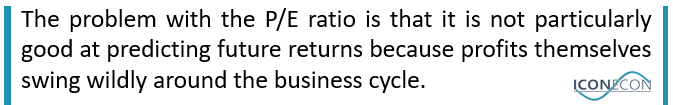Financial MarketsIntro: Valuation measures are only as useful as their ability to predict subsequent market returns. The valuations metrics that smooth out the short-term effects of the business cycle on profit margins tends to have the best track record of predicting future returns. For this reason, cyclically-adjusted measure, as well as the simple price-to-sales ratio, tend to be much more useful then the P/E ratio.

Useful Metrics

Dividend Yield: The dividend yield is the most intuitive valuation measure to use as it relates directly to equity returns. When dividend yields are high, it is intuitive that long-term equity returns will be high even if valuations do not rise because investors will receive a high dividend income stream. A high dividend yield also gives greater prospects for valuation increases.

Cyclically-Adjusted Dividend Yield: Dividend payouts can vary wildly around the economic cycle. At present for example, US companies are paying out very high dividends relative to earnings, sales, book values, cash flows, etc. The current dividend yield of 2.0x (price-to-dividend ratio of ~50x) gives an artificially favourable picture of how expensive US stocks are. In order to smooth out the impact of temporarily high or low dividend payments, we can take an average of dividends over say 10 years. This typically improves the dividend yield as a valuation measure.

Cyclically-Adjusted P/E Ratio (CAPE): Because of the fluctuations in earnings around the business cycle, investors often use the CAPE, which smooths out earnings my taking a 10-year moving average as a way of removing the impact of temporarily weak or strong earnings. This measure therefore has a much stronger correlation with subsequent returns.

Price-to-Sales Ratio: Another option is to look at the price-to-sales ratio, which has a similarly close correlation with subsequent market returns. The reason is that profit margins tend to mean revert over time and this measure strips out the impact of temporarily strong or weak profits. At present, for example, the S&P500 P/E ratio is only slightly above its long-term average, whereas the P/S ratio is close to its all-time high. The reason is that profits margins are currently near record highs, and because they have a tendency to mean revert over time, the P/S tends to be more reflective of how expensive stocks really are.

Market Capitalization Relative-to-GDP: The so-called Warrant Buffet indicator has a very strong correlation with subsequent equity market returns. The reason being that dividends cannot grow faster than nominal GDP indefinitely, and vice versa, and tend to mean revert over the long term, and so GDP itself is the most useful denominator to use.

Price-to-Book Ratio: The P/B ratio is also useful, although suffers from the problem of value traps, where equities may seem cheap as the ratio may be low but the ability of companies to turn their net assets into earnings and dividends may be low. Generally speaking the P/B ratio is most useful for analysing the financial sector.Not-So-Useful Metrics

The most common valuation measure is the P/E ratio and investors like this metric because it is intuitive. The problem is, it is not particularly good at predicting future returns, the reason being that profits themselves swing wildly around the business cycle. During recessions, earnings tend to collapse temporarily, making equities seem artificially expensive based on long-term earnings and dividend potential. This was the case during 2010 when US equities rallied but earnings were yet to recover.

Similarly, profits are now extremely high following the economic recovery, making equities look artificially cheap compared to other metrics that have stronger correlations with subsequent returns. An additional problem is that investors do not actually receive earnings, but dividends instead, and so in countries with poor corporate governance earnings can be overstated and therefore the dividends do not materialize as expected.

Investors often devise valuation metrics that fit their narrative but have very little use in predicting the future. One of the most flagrant examples is comparing the dividend yield or earnings yield on equities (earnings as a percentage of the equity price) to the yield to maturity on bonds. If the equity index dividend yield is higher than the 10-year bond yield, for example, it is often claimed that equities are the better option. The problem, however, is that this may just mean than bonds are expensive rather than saying anything about how cheap stocks are.